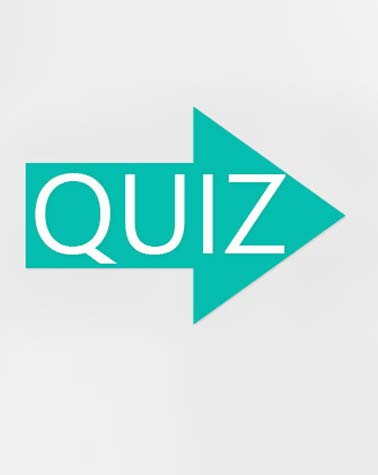# Chapter 4: Decimal Operations

Approved & Edited by ProProfs Editorial Team
The editorial team at ProProfs Quizzes consists of a select group of subject experts, trivia writers, and quiz masters who have authored over 10,000 quizzes taken by more than 100 million users. This team includes our in-house seasoned quiz moderators and subject matter experts. Our editorial experts, spread across the world, are rigorously trained using our comprehensive guidelines to ensure that you receive the highest quality quizzes.
| By Boleszczak
B
Boleszczak
Community Contributor
Quizzes Created: 1 | Total Attempts: 171
Questions: 10 | Attempts: 176SettingsQuestions are from Person C. M. P. Curriculum focusing on 6th grade operations of decimals.

• 1.

### True or False, Dan had the more money on Wednesday.

• A.

True

• B.

False

A. True
Explanation
Dan had more money on Wednesday because the question states that the answer is true.

Rate this question:

• 2.

### Dave accumulates the same amount of money every week. How much money should he expect to have after 3 and 1/2 weeks?

• A.

\$5.74

• B.

\$5.72

• C.

\$5.71

• D.

\$5.69

C. \$5.71
Explanation
Dave accumulates the same amount of money every week. After 3 and 1/2 weeks, he would have accumulated the same amount of money for 3 weeks and half of the amount for the 4th week. Therefore, the total amount of money he should expect to have after 3 and 1/2 weeks is the sum of 3 times the amount he accumulates in a week and half of the amount he accumulates in a week. The correct answer of \$5.71 is the closest option to this calculation.

Rate this question:

• 3.

### Who accumulated the most money Monday through Friday? By how much?

• A.

Dave

• B.

4 cents

• C.

Dan

• D.

8 cents

A. Dave
D. 8 cents
Explanation
Dave accumulated the most money Monday through Friday, by a difference of 8 cents compared to Dan.

Rate this question:

• 4.

### Find the exact sum for 0.52 + 1.2

1.72
Explanation
The given question asks for the exact sum of 0.52 and 1.2. The sum of these two numbers is 1.72.

Rate this question:

• 5.

### Find the exact difference for 4.4 - 1.29

3.11
Explanation
The given question asks for the exact difference between 4.4 and 1.29. To calculate the difference, we subtract 1.29 from 4.4. This gives us a result of 3.11. Therefore, the correct answer is 3.11.

Rate this question:

• 6.

### Find the exact product for 3.2 X 4.1

13.12
Explanation
The given answer, 13.12, is the exact product of multiplying 3.2 by 4.1.

Rate this question:

• 7.

### Find the quotient for 4.98 divided by 3.23 and round to the nearest hundredths place.

1.54
Explanation
The quotient for 4.98 divided by 3.23 is 1.54.

Rate this question:

• 8.

### How much gasoline did Paul buy on the trip?

61.0001 gallons, 61.0001
Explanation
Paul bought 61.0001 gallons of gasoline on the trip.

Rate this question:

• 9.

### How much money did Paul spend on gasoline for the entire trip?

\$213.50, \$213.51
• 10.

### Paul's odometer read 23,451.1 miles when he pulled out of his driveway. Then, when returning home it read 24,809.4 miles. How many miles did he travel on his trip?

1,358.3 miles
Explanation
Paul's odometer read 23,451.1 miles when he pulled out of his driveway and 24,809.4 miles when he returned home. To find out how many miles he traveled on his trip, we need to subtract the initial reading from the final reading. Therefore, the calculation would be 24,809.4 - 23,451.1 = 1,358.3 miles.

Rate this question:

Related TopicsBack to top